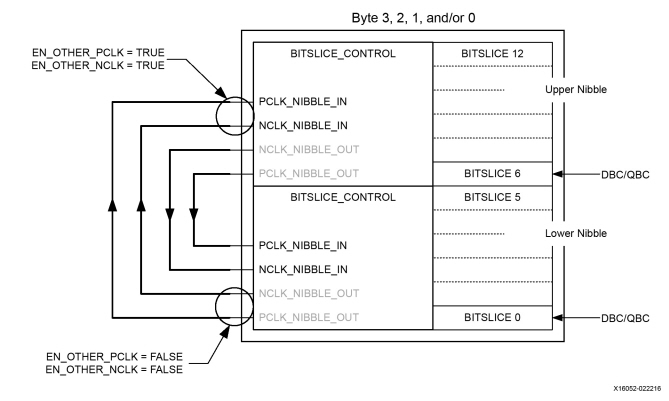# Inter-Nibble Clocking

## UltraScale Architecture SelectIO Resources User Guide (UG571)

Document ID
UG571
Release Date
2022-08-31
Revision
1.14 English

Each nibble has a possible clock input into BITSLICE_0. Two neighboring nibbles can share one of these clock’s inputs and increase the number of possible data inputs by joining together as a byte (This Figure).

One nibble passes the clock from its BITSLICE_0 input to the other nibble through dedicated inter-nibble clock routing from P(N)CLK_NIBBLE_OUT to the clock inputs at the other nibble P(N)CLK_NIBBLE_IN. This routing is enabled through attributes (EN_OTHER_P(N)_CLK) set at the BITSLICE_CONTROLs of both nibbles joined together as byte.

 X-Ref Target - Figure 2-68Using This Figure as an example, assuming the lower nibble's BITSLICE_0 is setup as a clock input (DATA_TYPE = DATA_AND_CLOCK) and the upper nibble BITSLICE_0 is used as data input, the attributes of both nibbles should be set as follows:

Upper nibble

EN_OTHER_PCLK = TRUE

EN_OTHER_NCLK = TRUE

Lower nibble

EN_OTHER_PCLK = FALSE

EN_OTHER_NCLK = FALSE

The clock passes through the lower BITSLICE_0 to the P(N)CLK_NIBBLE_OUT and into the upper nibble P(N)CLK_NIBBLE_IN inputs for clocking of the bit slices in the upper nibble.

When BITSLICE_0 of the upper nibble is used as a clock input, pass the clock to the lower nibble by using the P(N)CLK_NIBBLE_OUT pins of the upper nibble and the P(N)CLK_NIBBLE_IN pins of the lower nibble, and the attributes should be set as follows:

Upper nibble

EN_OTHER_PCLK = FALSE

EN_OTHER_NCLK = FALSE

Lower nibble

EN_OTHER_PCLK = TRUE

EN_OTHER_NCLK = TRUE

Note:   It is possible to enable inter-nibble clock routing in both directions so that bit slices in both nibbles can be used by one of the clocks connected to the byte. In other words, it is possible to capture data in the lower nibble at the clock applied to the upper nibble BITSLICE_0 while at the same time data can be captured in the upper nibble at the clock connected to the lower nibble BITSLICE_0.

TIP:   When using multiple nibbles in a design, always connect the inter-nibble clocks, as shown in This Figure. When inter-nibble clocking is necessary, enable or disable an attribute.